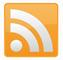Search this site

Custom Search

# Doing arithmetic with dates using Excel

Excel makes it easy to calculate with dates, but many are unfamiliar with its power.

Summary

• Excel holds dates as whole numbers, starting with 1=1 January 1900.
• That allows you to subtract dates to calculate the number of days between those dates.
• This makes it easy to calculate the internal rate of return.
• I also explain absolute and relative cell references.
• This was my second article for the UK Shareholders Association on monitoring investments with Excel.

Posted 22 March 2023

This was my second article for the UK Shareholders Association on monitoring investments with Excel.

For many reasons you often need to calculate how long you have owned an investment. Excel makes doing such calculations very easy, but I think many users are unaware of what it can do.

I also explain absolute and relative cell references. You can read it below.

## Monitoring investments with Excel – Part 2

In Part 1 I explained how to calculate an internal rate of return (IRR) using Excel, and promised that Part 2 would cover doing arithmetic with dates.

Before doing that, it is worth mentioning that in IRR calculations years don’t have to be whole numbers. In Part 1, where the investment had grown by 90%, I used the formula below to calculate the IRR:

R = 1.9(1/N) – 1

In this formula, N does not have to be a whole number of years. For example, if you have owned something for only six months, then N = 0.5 and the formula works just fine for calculating an annual IRR. Similarly, if you have owned a share for 30 months, then N = 2.5.

### How long have you owned a share?

I am writing this article on 19 December 2021. If you bought a share on 8 April 2013, how long have you owned it in years, writing the year as a decimal (to use in the above formula)?

Working that out by hand becomes tedious very quickly, especially if you value your shares, and compute the IRR, at the end of each month as I do. However, Excel makes it very easy.

In Excel, every date is held by the program as a whole number of days starting with 1 January 1900 which has the value 1.

The PDF file of the spreadsheet with normal appearance shows this. Accordingly, 8 April 2013 has the day-count of 41,372 and 19 December 2021 has the day-count of 44,549. The difference between those values, 3,177 is the number of days that you have owned the share.

You could calculate the IRR as the growth rate per day and then convert that into an annual rate (using the compound interest formula) but it is easier to convert 3,177 days into years. It is approximately 8.70 years.

I always work on the basis that each year is 365.25 days long, rather than trying to identify specific leap years. As well as being easier, that also corresponds with reality. The Earth does not take an integral number of days to orbit the Sun!

You can then list your portfolio, as I have done in the PDF file, working out the IRR line by line for each holding.

### Relative and absolute cell references

When you look at the spreadsheet in formula view, you will see that in cell H42 the formula is written as:

=(+F42/D42)^(1/((\$F\$36-C42)/365.25))-1

In the formula, look closely at \$F\$36. This is an absolute cell reference.

Normally in Excel references to other cells are relative to the cell in which the formula is located. For example at the beginning of the formula F42 is not really specifying cell F42. Instead, it is specifying the cell which is two spaces to the left of the cell containing the formula, and on the same row as the cell containing the formula.

Accordingly, if you copy cell H42 and paste it into cell H43, the formula now references cell F43 which is what you want. Similarly, if I pasted the formula in cell H42 into cell L66, the beginning of the formula would then reference cell J66.

This default approach works fine in most cases. However, you sometimes want a reference in a formula to point to a fixed cell, even if the formula is copied and pasted somewhere else. For example, in the above formula the \$F\$36 is pointing at the cell which contains today’s date.

When this formula is copied from cell H42 to cell H43 (or to any other cell) you still want it to reference today’s date in cell F36 and not some other cell. The two \$ signs in \$F\$36 tell Excel that this is an absolute reference, so even if the formula is copied elsewhere, it should still reference cell F36.

In passing, the reason there are two \$ signs is that each part of the formula can independently be either absolute or relative. Depending on what you want to happen when a reference like M8 is copied and pasted, your formula could be M8 or \$M8 or M\$8 or \$M\$8. Most of the time my formulas use either wholly relative or wholly absolute cell references, but occasionally this extra flexibility is useful.

The next time I will look at calculating the internal rate of return when you bought your shareholding in stages and not all at once.

Mohammed Amin MBE FRSA MA FCA AMCT CTA(Fellow)
Editor's note: although Amin is a member of UKSA’s Policy Team, he is writing in a personal capacity.